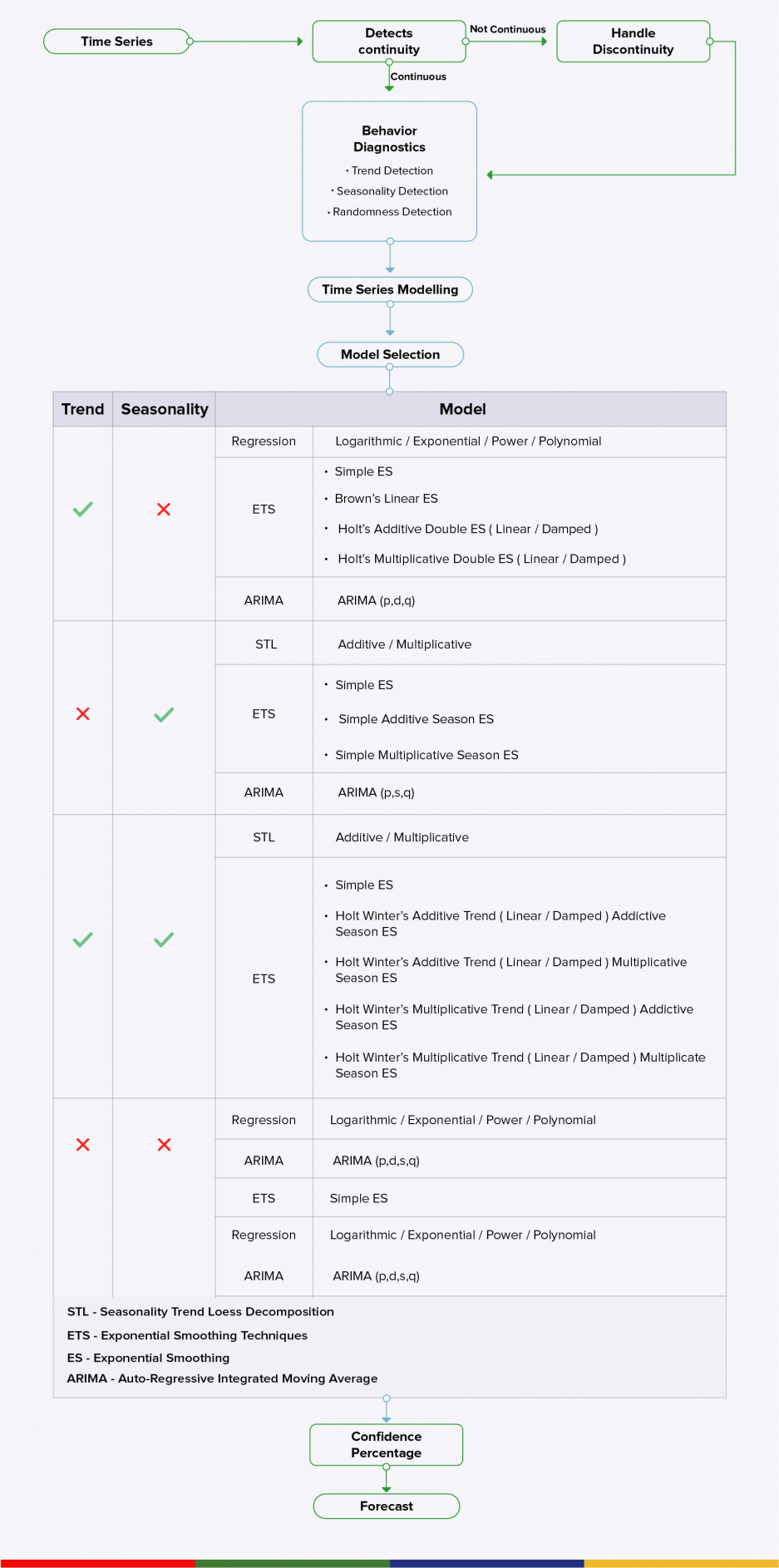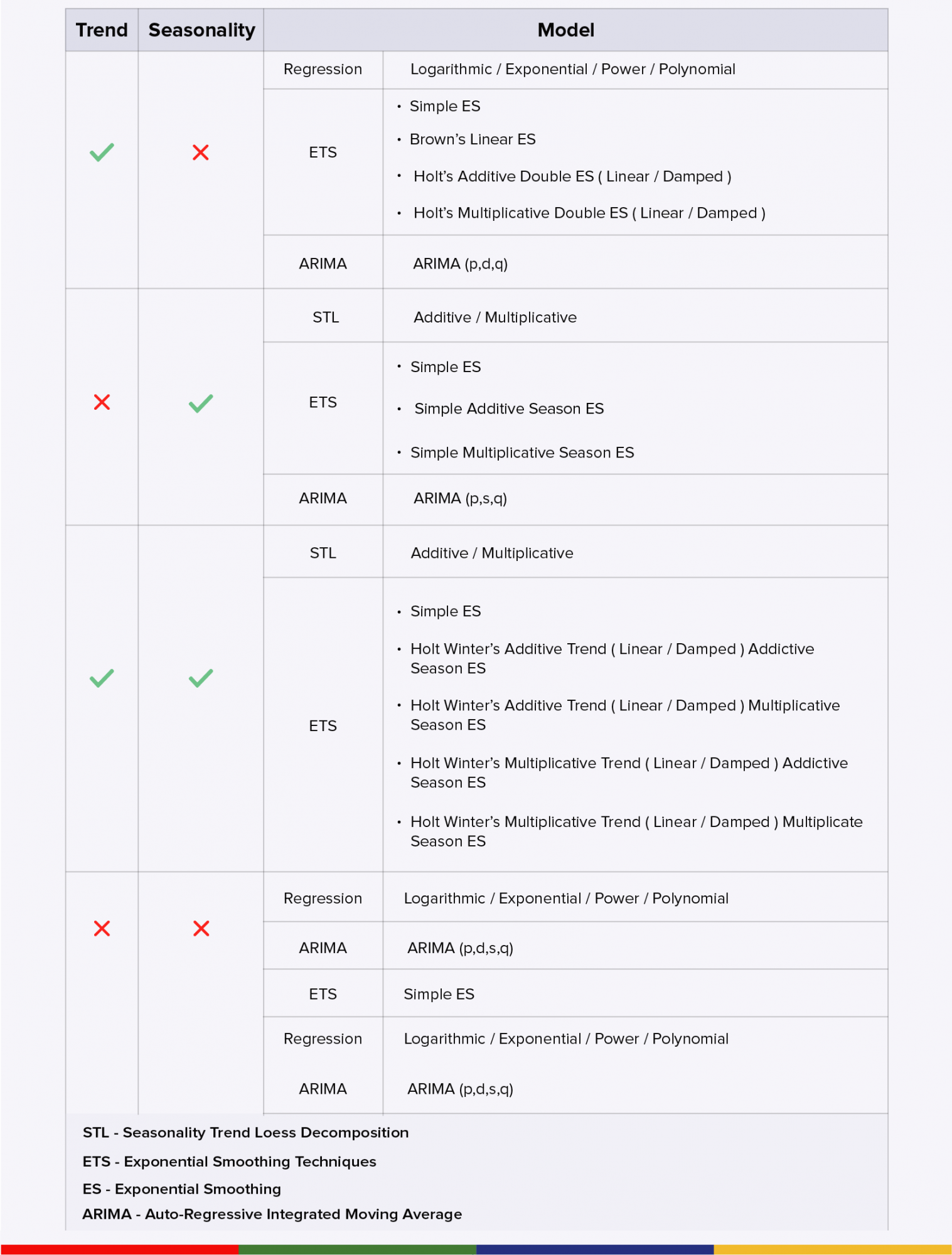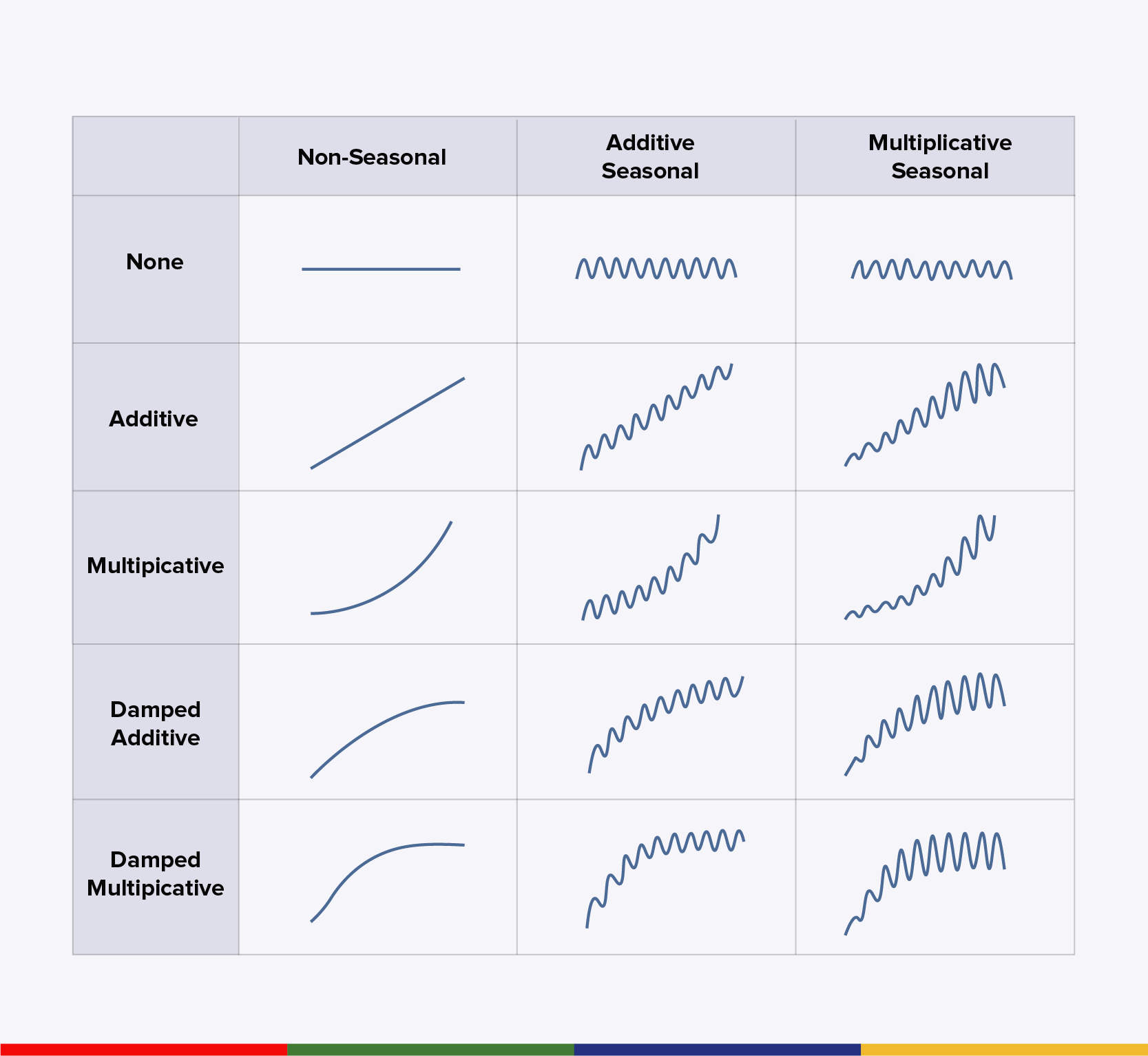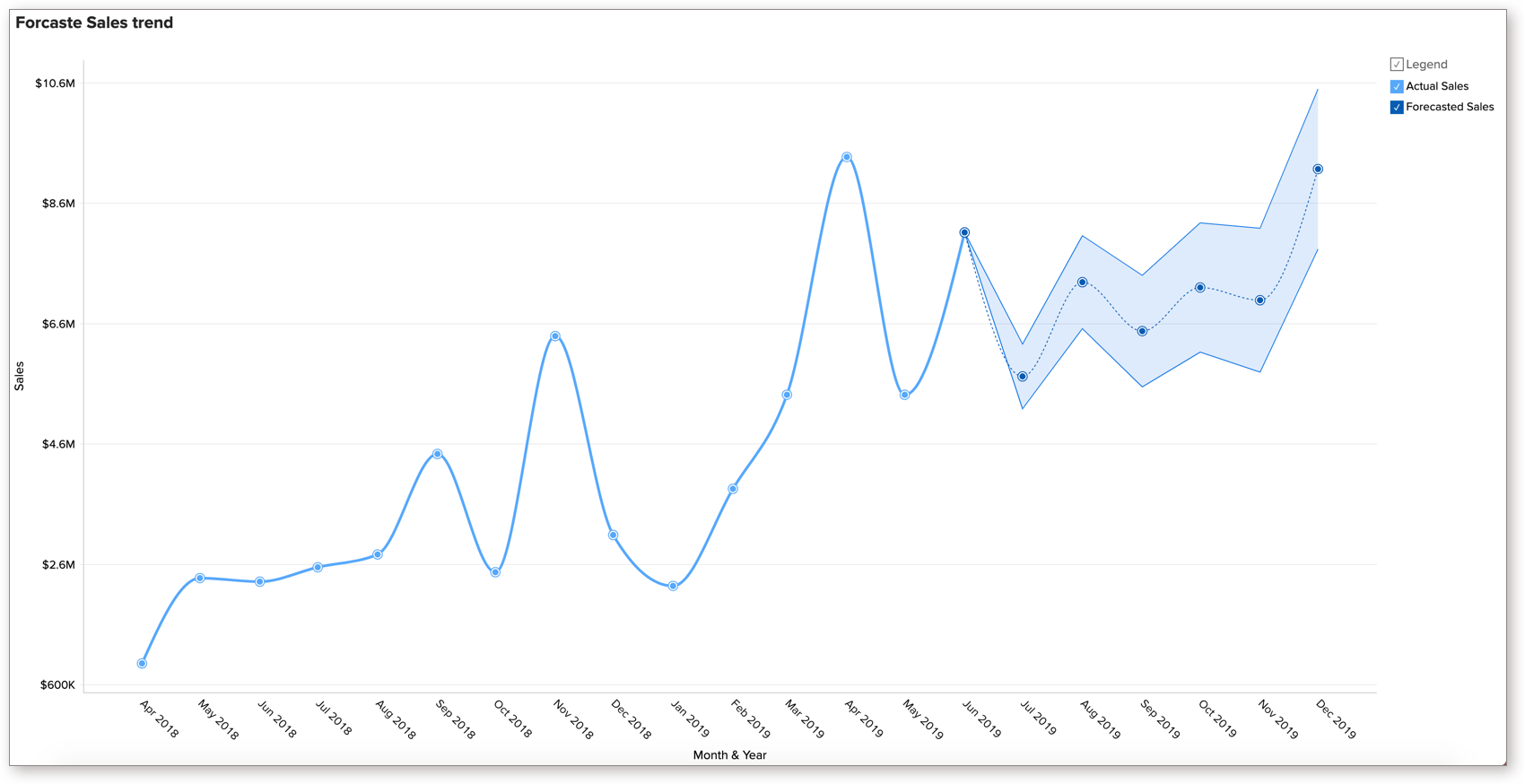# How Forecasting Works

Zoho Advanced Analytics offers a powerful forecast engine that predicts future data points based on past data. The forecast engine uses various techniques to analyze your timeseries data deeply, identify the pattern in your data and selects the best forecast model to predict the future accurately.

## Introduction

The forecast engine uses various techniques to analyze your timeseries data deeply, identify the pattern in your data, chooses the best forecast model based on the identified pattern, and predict the future accurately. The forecasting engine offers a range of customizing options such as the number of units to be forecasted, the number of data points to be ignored in the past data and the confidence interval within which the data is most likely to fall.

The following flow illustrates how the forecast engine works.## Timeseries

Zoho Advanced Analytics allows you to forecast any timeseries data i.e., any metric collected at equal time intervals. You can forecast a report which has at least one aggregate column plotted against a continuous timeseries. Zoho Advanced Analytics forecast engine requires a minimum of six continuous data points in the timeseries to analyze and forecast data. The more data points your report has, the better the forecast result will be.

In case there are missing values in your data, Zoho Advanced Analytics will fill the values for the missing data points. To auto-fill values, the forecast engine will get the average of the preceding and the succeeding data point values to fill the places.

Note: If the missing values are more than 40% of the original data, the forecast engine stops the process to avoid inaccurate forecasts.

## Data Analysis

Now the forecast engine gets the data points for a continuous time series. It will learn the presence of trend, seasonality, and randomness in the timeseries data. These facts are identified to decide on the model for forecasting.

### Trend

Trend refers to a uniform increase or decrease in the series over time. The forecast engine detects the presence of a trend in the data series using the Regression model. If the line drawn using the Regression model has a slope, then a trend is present in the data. If the line drawn is straight, there is no trend in data.

### Seasonality

Seasonality is a predictable repeating value in an estimated frequency. This is calculated using the spectral analysis

• Additive - Here, the contributions of the components are almost the same.
• Multiplicative - Here, at least some component contributions are in multiplies.

### Randomness

Randomness is random points without any identified pattern among the data series. This is also called as an error.

## Model Selection

Depending on the identified patterns in the data (presence of Trend, Seasonality, and Randomness), the forecast engine first auto-selects a set of forecast models for your data series. And finally, it selects the model with the best result as the forecast model for your data.

The following screen lists the forecast models that will be applied over the corresponding patterns identified.The following are the forecast models used to forecast the data series. Each model will have a set of models within.

### Regression

Regression is based on the data science model. This studies the behavior of the data series and finds parameters to fit the models. Once it studies the patterns among the random series, different regression models such as linear, logarithmic, exponential, power and polynomial (up to degree 7) will be tried over them. These models will plot a line along with the existing data. To identify the best model, the forecast engine will use the R^2 method. The model with the highest R-squared  is selected as the best result for the Regression model.

### Seasonality Trend Loess Decomposition (STL)

With Seasonal Trend Loess decomposition, we get the trend, season, and error components. The seasonal component is assumed to be repetitive. The trend, season, and error are then combined either by additive or by multiplicative method for calculating the future values. Random points smoothening will also be taken care of in this model.

Trend + Seasonality + Randomness

The Multiplicative model will multiply trend, seasonality and random points together.

Trend * Seasonality * Randomness

The best model in STL is selected using the Root Mean Square Error (RMSE) method.

### Exponential Smoothing Technique / Explicit handling of Trend and Seasonality (ETS)

The forecast engine employs a set of exponential smoothing techniques based on your data. In this model, recent values influence the forecast more.

The following screen illustrates the possible combinations of additive/multiplicative/dampening models over trend and seasonality.The following list briefs the ETS models:

• Single/Simple Exponential Smoothing (ES) - This uses a single smoothing parameter (level) or a data series without any observable trend and seasonality.
• Double Exponential Smoothing - This is an extended simple ES with another smoothing parameter (trend) to handle the trend in data series.
• Brown's Linear ES - In this model, both the parameters smoothens the trend.
• Holt's Additive Double ES* - This model is for a series with the additive trend.
• Holt's Multiplicative Double ES* - This model is for a series with the multiplicative trend.

* Holt's Additive/ Multiplicative Double ES employs different models for linear trend and damped trend.

• Triple Exponential Smoothing (Holt Winter's ES) - This model has a third smoothing parameter (Seasonality) to support for seasonal variations.

The best model out of the ETS models is selected using the Akaike information criterion (AIC)method.

### Autoregressive Integrated Moving Average (ARIMA)

This is a conventional approach to time series modeling that relies on successive transformations until white noise or the original signal of the underlying data generation process is extracted. It employs auto-regression processes and moving averages to propagate the nature of the series, along with probable errors. The ARIMA model is best suited for a random data series, with no proper trend and seasonality components.

## Verifying Forecast Model

Now the forecast engine will compare the result of each model employed and identifies the best model to forecast the chart. This is done using the Akaike information criterion (AIC) primarily. In case if two models give similar results, then the Bayesian information criterion (BIC) method will be used to pick the final forecast method.

## Confidence Percentage

In case of a line chart, instead of a single value, the forecast engine can predict a range in which the future data point is likely to occur. Confidence Limit is estimated using normal probability distribution. You can choose to predict a probability interval of 70% to 95% confidence, within which the data point is most likely to occur. You can also choose to set this as none.

## Final Forecast

Zoho Advanced Analytics will use the best model selected to predict future value. You can also predict the probability interval within which the data point is most likely to occur.